Next: 7.3 Five-Stage CML Ring Up: 7. Simulation Results Previous: 7.1.2 Silicon-Germanium HBT

# 7.2 Five-Stage CMOS Ring Oscillator

Amongst the simplest digital circuits is the CMOS inverter as shown in Fig. 6.15. It consists of two complementary MOS transistors, an NMOS and a PMOS. During the two steady states only the leakage current flows through the devices and power dissipation is negligible. However, when switching from one state to the other both transistors conduct a much higher current which determines the power dissipation.

The measured delay time depends on the shape of the input curve and on the load at the output. Two conditions should hold: first, the inverter should only delay the signal without distorting it and secondly, the load at the output should be equal to the input impedance of the inverter. These conditions are provided by ring oscillators which are frequently used to measure the delay time of digital circuits . A ring oscillator consists of n = 2 . k + 1 inverters and the inverter delay time results to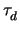=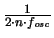(7.6)

with fosc being the oscillation frequency.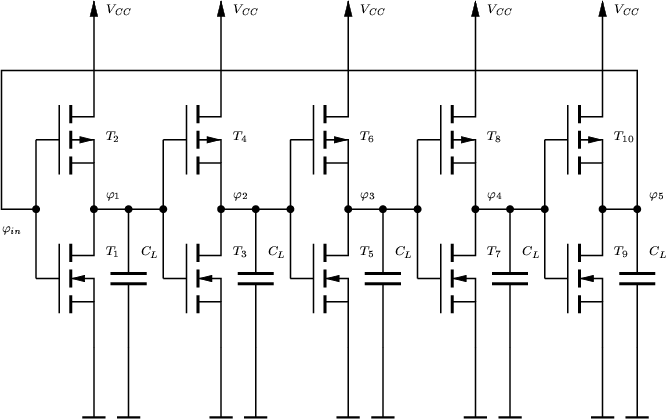A five-stage ring oscillator circuit is shown in Fig. 7.11. For both transistors a device width of W = 1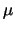m was assumed. Normally, to achive equal noise margins, a ratio of Wp/Wn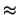2.5 is used to compensate for the poorer performance of the PMOS transistor . To model the influence of the interconnect circuitry, an additional load capacity of 5 fF was used. The operating point calculation of this circuit provided no problems. However, to force the circuit into a predefined initial state, the input voltage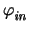of the first inverter was set to zero during operating point calculation.

Two different ring oscillators have been simulated, one with the long-channel transistors, the other with the short-channel transistors. The respective simulation results are shown in Fig. 7.12 and Fig. 7.13. For the long-channel transistors, the simulation results obtained with the DD and HD transport models agree so closely, that in the graph no differences are visible. However, when using the short-channel devices, the differences are significant. This is due to the larger currents resulting from the HD transport model. The charging and discharging times of an inverter chain is given as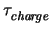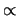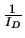. (7.7)

The simulated inverter delay times are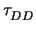30 ns and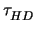26 ns giving a difference of about 15 %. In Fig. 3.16 and Fig. 3.18 the HD currents are approximately 30 % and 5 % higher for the NMOS and the PMOS transistor, respectively. The average of these values (17.5 %) nicely corresponds to the simulated delay time difference of 15 %.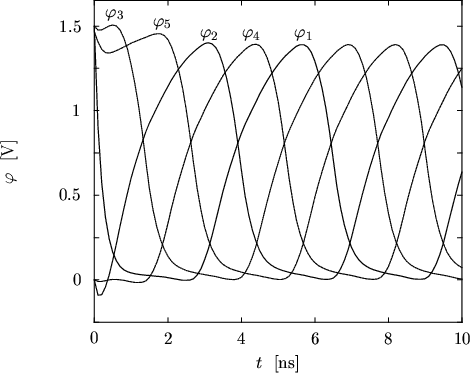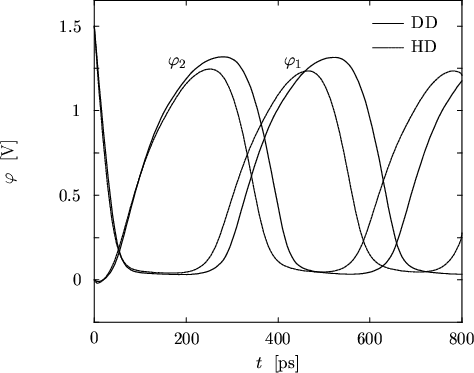Next: 7.3 Five-Stage CML Ring Up: 7. Simulation Results Previous: 7.1.2 Silicon-Germanium HBT
Tibor Grasser
1999-05-31